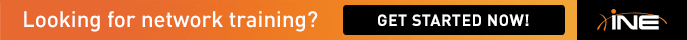# BGP dampening formula

Hello,

Can anyone explain from where did we get this formula -P(t) = P(0)/2^(t/Half_Life) ?

As per Cisco documentation, the formula is:

Max-Penalty= reuse-limit *2^(max-suppress-time/half-life)

Seems like the Workbook is replacing Max-penalty with P(0) (Suppress-penalty), which are two different values, and the difference can be seen with the show ip bgp dampening parameters...

Am I missing something?

• The P(t) = P(0)/2^(t/Half_Life) is the equation for exponential decay.

Pt=P0^(t/half life)

The formula you are referring to from the Cisco documentation is for the maximum penalty.

Max-Penalty= reuse-limit *2^(max-suppress-time/half-life)

Taking it a step further, use a Half Life Calculator (you can find one on Google) to help you understand the formula and how the solution guide calculated the half-life of 4.

Here is the breakdown:

P(T) = 750 =  is the quantity that still remains after a time [Default reuse limit]

P(0) = 2000 = the initial quantity  [Default Suppress Limit]

Time = 5  [The value from the question “the advertisement should resume in 5 minutes.”]

If you enter them in the half-life calculator you get 3.5334752630571

As part of the solution, they round up to 4.

Next, you can calculate the Max-Penalty based on the formula below:

“Max-Penalty= reuse-limit *2^(max-suppress-time/half-life)”

Max-Penatly =  750 * 2^( max-suppress-time /4)

Max-Suppress-time the default is 4 times the half-life = 16

Max-Penatly =  750 * 2^( 16 /4)

Max-Suppress penalty = 12000

bgp dampening 4 750 2000 16

4  is half life

750 is default reuse limit

2000 is default suppress limit

Max-Suppress default is 4 times the half-life, which is 16

• Can anyone explain from where did we get this formula -P(t) = P(0)/2^(t/Half_Life) ?

See the following post - http://ieoc.com/forums/p/17521/152933.aspx I think this will cover your questions!